Calculus Volume 2

# 4.2Direction Fields and Numerical Methods

Calculus Volume 24.2 Direction Fields and Numerical Methods

### Learning Objectives

• 4.2.1 Draw the direction field for a given first-order differential equation.
• 4.2.2 Use a direction field to draw a solution curve of a first-order differential equation.
• 4.2.3 Use Euler’s Method to approximate the solution to a first-order differential equation.

For the rest of this chapter we will focus on various methods for solving differential equations and analyzing the behavior of the solutions. In some cases it is possible to predict properties of a solution to a differential equation without knowing the actual solution. We will also study numerical methods for solving differential equations, which can be programmed by using various computer languages or even by using a spreadsheet program, such as Microsoft Excel.

### Creating Direction Fields

Direction fields (also called slope fields) are useful for investigating first-order differential equations. In particular, we consider a first-order differential equation of the form

$y′=f(x,y).y′=f(x,y).$

An applied example of this type of differential equation appears in Newton’s law of cooling, which we will solve explicitly later in this chapter. First, though, let us create a direction field for the differential equation

$T′(t)=−0.4(T−72).T′(t)=−0.4(T−72).$

Here $T(t)T(t)$ represents the temperature (in degrees Fahrenheit) of an object at time $t,t,$ and the ambient temperature is $72°F.72°F.$ Figure 4.6 shows the direction field for this equation.

Figure 4.6 Direction field for the differential equation $T′(t)=−0.4(T−72).T′(t)=−0.4(T−72).$ Two solutions are plotted: one with initial temperature less than $72°F72°F$ and the other with initial temperature greater than $72°F.72°F.$

The idea behind a direction field is the fact that the derivative of a function evaluated at a given point is the slope of the tangent line to the graph of that function at the same point. Other examples of differential equations for which we can create a direction field include

$y′=3x+2y−4y′=x2−y2y′=2x+4y−2.y′=3x+2y−4y′=x2−y2y′=2x+4y−2.$

To create a direction field, we start with the first equation: $y′=3x+2y−4.y′=3x+2y−4.$ We let $(x0,y0)(x0,y0)$ be any ordered pair, and we substitute these numbers into the right-hand side of the differential equation. For example, if we choose $x=1andy=2,x=1andy=2,$ substituting into the right-hand side of the differential equation yields

$y′=3x+2y−4=3(1)+2(2)−4=3.y′=3x+2y−4=3(1)+2(2)−4=3.$

This tells us that if a solution to the differential equation $y′=3x+2y−4y′=3x+2y−4$ passes through the point $(1,2),(1,2),$ then the slope of the solution at that point must equal $3.3.$ To start creating the direction field, we put a short line segment at the point $(1,2)(1,2)$ having slope $3.3.$ We can do this for any point in the domain of the function $f(x,y)=3x+2y−4,f(x,y)=3x+2y−4,$ which consists of all ordered pairs $(x,y)(x,y)$ in $ℝ2.ℝ2.$ Therefore any point in the Cartesian plane has a slope associated with it, assuming that a solution to the differential equation passes through that point. The direction field for the differential equation $y′=3x+2y−4y′=3x+2y−4$ is shown in Figure 4.7.

Figure 4.7 Direction field for the differential equation $y′=3x+2y−4.y′=3x+2y−4.$

We can generate a direction field of this type for any differential equation of the form $y′=f(x,y).y′=f(x,y).$

### Definition

A direction field (slope field) is a mathematical object used to graphically represent solutions to a first-order differential equation. At each point in a direction field, a line segment appears whose slope is equal to the slope of a solution to the differential equation passing through that point.

### Using Direction Fields

We can use a direction field to predict the behavior of solutions to a differential equation without knowing the actual solution. For example, the direction field in Figure 4.7 serves as a guide to the behavior of solutions to the differential equation $y′=3x+2y−4.y′=3x+2y−4.$

To use a direction field, we start by choosing any point in the field. The line segment at that point serves as a signpost telling us what direction to go from there. For example, if a solution to the differential equation passes through the point $(0,1),(0,1),$ then the slope of the solution passing through that point is given by $y′=3(0)+2(1)−4=−2.y′=3(0)+2(1)−4=−2.$ Now let $xx$ increase slightly, say to $x=0.1.x=0.1.$ Using the method of linear approximations gives a formula for the approximate value of $yy$ for $x=0.1.x=0.1.$ In particular,

$L(x)=y0+f′(x0)(x−x0)=1−2(x−0)=1−2x.L(x)=y0+f′(x0)(x−x0)=1−2(x−0)=1−2x.$

Substituting $x=0.1x=0.1$ into $L(x)L(x)$ gives an approximate $yy$ value of $0.8.0.8.$

At this point the slope of the solution changes (again according to the differential equation). We can keep progressing, recalculating the slope of the solution as we take small steps to the right, and watching the behavior of the solution. Figure 4.8 shows a graph of the solution passing through the point $(0,1).(0,1).$

Figure 4.8 Direction field for the differential equation $y′=3x+2y−4y′=3x+2y−4$ with the solution passing through the point $(0,1).(0,1).$

The curve is the graph of the solution to the initial-value problem

$y′=3x+2y−4,y(0)=1.y′=3x+2y−4,y(0)=1.$

This curve is called a solution curve passing through the point $(0,1).(0,1).$ The exact solution to this initial-value problem is

$y=−32x+54−14e2x,y=−32x+54−14e2x,$

and the graph of this solution is identical to the curve in Figure 4.8.

### Checkpoint4.7

Create a direction field for the differential equation $y′=x2−y2y′=x2−y2$ and sketch a solution curve passing through the point $(−1,2).(−1,2).$

### Media

Go to this website to see more about slope fields.

Now consider the direction field for the differential equation $y′=(x−3)(y2−4),y′=(x−3)(y2−4),$ shown in Figure 4.9. This direction field has several interesting properties. First of all, at $y=−2y=−2$ and $y=2,y=2,$ horizontal dashes appear all the way across the graph. This means that if $y=−2,y=−2,$ then $y′=0.y′=0.$ Substituting this expression into the right-hand side of the differential equation gives

$(x−3)(y2−4)=(x−3)((-2)2−4)=(x−3)(0)=0=y′.(x−3)(y2−4)=(x−3)((-2)2−4)=(x−3)(0)=0=y′.$

Therefore $y=−2y=−2$ is a solution to the differential equation. Similarly, $y=2y=2$ is a solution to the differential equation. These are the only constant-valued solutions to the differential equation, as we can see from the following argument. Suppose $y=ky=k$ is a constant solution to the differential equation. Then $y′=0.y′=0.$ Substituting this expression into the differential equation yields $0=(x−3)(k2−4).0=(x−3)(k2−4).$ This equation must be true for all values of $x,x,$ so the second factor must equal zero. This result yields the equation $k2−4=0.k2−4=0.$ The solutions to this equation are $k=−2k=−2$ and $k=2,k=2,$ which are the constant solutions already mentioned. These are called the equilibrium solutions to the differential equation.

Figure 4.9 Direction field for the differential equation $y′=(x−3)(y2−4)y′=(x−3)(y2−4)$ showing two solutions. These solutions are very close together, but one is barely above the equilibrium solution $x=−2x=−2$ and the other is barely below the same equilibrium solution.

### Definition

Consider the differential equation $y′=f(x,y).y′=f(x,y).$ An equilibrium solution is any solution to the differential equation of the form $y=c,y=c,$ where $cc$ is a constant.

To determine the equilibrium solutions to the differential equation $y′=f(x,y),y′=f(x,y),$ set the right-hand side equal to zero. An equilibrium solution of the differential equation is any function of the form $y=ky=k$ such that $f(x,k)=0f(x,k)=0$ for all values of $xx$ in the domain of $f.f.$

An important characteristic of equilibrium solutions concerns whether or not they approach the line $y=ky=k$ as an asymptote for large values of $x.x.$

### Definition

Consider the differential equation $y′=f(x,y),y′=f(x,y),$ and assume that all solutions to this differential equation are defined for $x≥x0.x≥x0.$ Let $y=ky=k$ be an equilibrium solution to the differential equation.

1. $y=ky=k$ is an asymptotically stable solution to the differential equation if there exists $ε>0ε>0$ such that for any value $c∈(k−ε,k+ε)c∈(k−ε,k+ε)$ the solution to the initial-value problem
$y′=f(x,y),y(x0)=cy′=f(x,y),y(x0)=c$

approaches $kk$ as $xx$ approaches infinity.
2. $y=ky=k$ is an asymptotically unstable solution to the differential equation if there exists $ε>0ε>0$ such that for any value $c∈(k−ε,k+ε)c∈(k−ε,k+ε)$ the solution to the initial-value problem
$y′=f(x,y),y(x0)=cy′=f(x,y),y(x0)=c$

never approaches $kk$ as $xx$ approaches infinity.
3. $y=ky=k$ is an asymptotically semi-stable solution to the differential equation if it is neither asymptotically stable nor asymptotically unstable.

Now we return to the differential equation $y′=(x−3)(y2−4),y′=(x−3)(y2−4),$ with the initial condition $y(0)=0.5.y(0)=0.5.$ The direction field for this initial-value problem, along with the corresponding solution, is shown in Figure 4.10.

Figure 4.10 Direction field for the initial-value problem $y′=(x−3)(y2−4),y(0)=0.5.y′=(x−3)(y2−4),y(0)=0.5.$

The values of the solution to this initial-value problem stay between $y=−2y=−2$ and $y=2,y=2,$ which are the equilibrium solutions to the differential equation. However, an initial-value problem starting with $-2 can never cross the equilibrium solutions $y=2y=2$ and $y=-2y=-2$. Therefore, since $y2-4<0y2-4<0$ and for $x>3,y'=(x-3)(y2-4)<0x>3,y'=(x-3)(y2-4)<0$, $yy$ is decreasing and therefore approaches $y=-2y=-2$. Therefore, $y=-2y=-2$ is an asymptotically stable solution to the differential equation.

What happens when the initial value is below $y=−2?y=−2?$ This scenario is illustrated in Figure 4.11, with the initial value $y(0)=−3.y(0)=−3.$

Figure 4.11 Direction field for the initial-value problem $y′=(x−3)(y2−4),y(0)=−3.y′=(x−3)(y2−4),y(0)=−3.$

We can see that for initial values $y<-2y<-2$ for $x>3,y'(x-3)(y2-4)>0x>3,y'(x-3)(y2-4)>0$ and $yy$ is increasing and therefore approaches $y=-2y=-2$. This reaffirms that $y=-2y=-2$ is an asymptotically stable solution to the differential equation.

### Example 4.8

#### Stability of an Equilibrium Solution

Create a direction field for the differential equation $y′=(y−3)2(y2+y−2)y′=(y−3)2(y2+y−2)$ and identify any equilibrium solutions. Classify each of the equilibrium solutions as stable, unstable, or semi-stable.

#### Analysis

It is possible to find the equilibrium solutions to the differential equation by setting the right-hand side equal to zero and solving for $y.y.$ This approach gives the same equilibrium solutions as those we saw in the direction field.

### Checkpoint4.8

Create a direction field for the differential equation $y′=(x+5)(y+2)(y2−4y+4)y′=(x+5)(y+2)(y2−4y+4)$ and identify any equilibrium solutions. Classify each of the equilibrium solutions as stable, unstable, or semi-stable.

### Euler’s Method

Consider the initial-value problem

$y′=2x−3,y(0)=3.y′=2x−3,y(0)=3.$

Integrating both sides of the differential equation gives $y=x2−3x+C,y=x2−3x+C,$ and solving for $CC$ yields the particular solution $y=x2−3x+3.y=x2−3x+3.$ The solution for this initial-value problem appears as the parabola in Figure 4.13.

Figure 4.13 Euler’s Method for the initial-value problem $y′=2x−3,y(0)=3.y′=2x−3,y(0)=3.$

The red graph consists of line segments that approximate the solution to the initial-value problem. The graph starts at the same initial value of $(0,3).(0,3).$ Then the slope of the solution at any point is determined by the right-hand side of the differential equation, and the length of the line segment is determined by increasing the $xx$ value by $0.50.5$ each time (the step size). This approach is the basis of Euler’s Method.

Before we state Euler’s Method as a theorem, let’s consider another initial-value problem:

$y′=x2−y2,y(−1)=2.y′=x2−y2,y(−1)=2.$

The idea behind direction fields can also be applied to this problem to study the behavior of its solution. For example, at the point $(−1,2),(−1,2),$ the slope of the solution is given by $y′=(−1)2−22=−3,y′=(−1)2−22=−3,$ so the slope of the tangent line to the solution at that point is also equal to $−3.−3.$ Now we define $x0=−1x0=−1$ and $y0=2.y0=2.$ Since the slope of the solution at this point is equal to $−3,−3,$ we can use the method of linear approximation to approximate $yy$ near $(−1,2).(−1,2).$

$L(x)=y0+f′(x0)(x−x0).L(x)=y0+f′(x0)(x−x0).$

Here $x0=−1,y0=2,x0=−1,y0=2,$ and $f′(x0)=−3,f′(x0)=−3,$ so the linear approximation becomes

$L(x)=2−3(x−(−1))=2−3x−3=−3x−1.L(x)=2−3(x−(−1))=2−3x−3=−3x−1.$

Now we choose a step size. The step size is a small value, typically $0.10.1$ or less, that serves as an increment for $x;x;$ it is represented by the variable $h.h.$ In our example, let $h=0.1.h=0.1.$ Incrementing $x0x0$ by $hh$ gives our next $xx$ value:

$x1=x0+h=−1+0.1=−0.9.x1=x0+h=−1+0.1=−0.9.$

We can substitute $x1=−0.9x1=−0.9$ into the linear approximation to calculate $y1.y1.$

$y1=L(x1)=−3(−0.9)−1=1.7.y1=L(x1)=−3(−0.9)−1=1.7.$

Therefore the approximate $yy$ value for the solution when $x=−0.9x=−0.9$ is $y=1.7.y=1.7.$ We can then repeat the process, using $x1=−0.9x1=−0.9$ and $y1=1.7y1=1.7$ to calculate $x2x2$ and $y2.y2.$ The new slope is given by $y′=(−0.9)2−(1.7)2=−2.08.y′=(−0.9)2−(1.7)2=−2.08.$ First, $x2=x1+h=−0.9+0.1=−0.8.x2=x1+h=−0.9+0.1=−0.8.$ Using linear approximation gives

$L(x)=y1+f′(x1)(x−x1)=1.7−2.08(x−(−0.9))=1.7−2.08x−1.872=−2.08x−0.172.L(x)=y1+f′(x1)(x−x1)=1.7−2.08(x−(−0.9))=1.7−2.08x−1.872=−2.08x−0.172.$

Finally, we substitute $x2=−0.8x2=−0.8$ into the linear approximation to calculate $y2.y2.$

$y2=L(x2)=−2.08x2−0.172=−2.08(−0.8)−0.172=1.492.y2=L(x2)=−2.08x2−0.172=−2.08(−0.8)−0.172=1.492.$

Therefore the approximate value of the solution to the differential equation is $y=1.492y=1.492$ when $x=−0.8.x=−0.8.$

What we have just shown is the idea behind Euler’s Method. Repeating these steps gives a list of values for the solution. These values are shown in Table 4.2, rounded off to four decimal places.

 $nn$ $00$ $11$ $22$ $33$ $44$ $55$ $xnxn$ $−1−1$ $−0.9−0.9$ $−0.8−0.8$ $−0.7−0.7$ $−0.6−0.6$ $−0.5−0.5$ $ynyn$ $22$ $1.71.7$ $1.4921.492$ $1.33341.3334$ $1.20461.2046$ $1.09551.0955$ $nn$ $66$ $77$ $88$ $99$ $1010$ $xnxn$ $−0.4−0.4$ $−0.3−0.3$ $−0.2−0.2$ $−0.1−0.1$ $00$ $ynyn$ $1.00041.0004$ $1.91641.9164$ $1.84141.8414$ $1.77461.7746$ $1.71561.7156$
Table 4.2 Using Euler’s Method to Approximate Solutions to a Differential Equation

### Theorem4.1

#### Euler’s Method

Consider the initial-value problem

$y′=f(x,y),y(x0)=y0.y′=f(x,y),y(x0)=y0.$

To approximate a solution to this problem using Euler’s method, define

$xn=x0+nhyn=yn−1+hf(xn−1,yn−1).xn=x0+nhyn=yn−1+hf(xn−1,yn−1).$
(4.2)

Here $h>0h>0$ represents the step size and $nn$ is an integer, starting with $1.1.$ The number of steps taken is counted by the variable $n.n.$

Typically $hh$ is a small value, say $0.10.1$ or $0.05.0.05.$ The smaller the value of $h,h,$ the more calculations are needed. The higher the value of $h,h,$ the fewer calculations are needed. However, the tradeoff results in a lower degree of accuracy for larger step size, as illustrated in Figure 4.14.

Figure 4.14 Euler’s method for the initial-value problem $y′=2x−3,y(0)=3y′=2x−3,y(0)=3$ with (a) a step size of $h=0.5;h=0.5;$ and (b) a step size of $h=0.25.h=0.25.$

### Example 4.9

#### Using Euler’s Method

Consider the initial-value problem

$y′=3x2−y2+1,y(0)=2.y′=3x2−y2+1,y(0)=2.$

Use Euler’s method with a step size of $0.10.1$ to generate a table of values for the solution for values of $xx$ between $00$ and $1.1.$

### Checkpoint4.9

Consider the initial-value problem

$y′=x3+y2,y(1)=−2.y′=x3+y2,y(1)=−2.$

Using a step size of $0.1,0.1,$ generate a table with approximate values for the solution to the initial-value problem for values of $xx$ between $11$ and $2.2.$

### Media

Visit this website for a practical application of the material in this section.

### Section 4.2 Exercises

For the following problems, use the direction field below from the differential equation $y′=−2y.y′=−2y.$ Sketch the graph of the solution for the given initial conditions.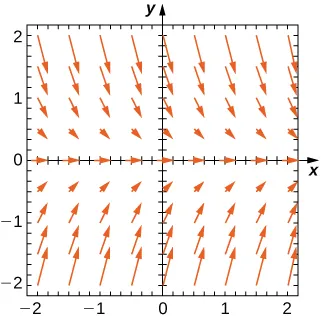66.

$y ( 0 ) = 1 y ( 0 ) = 1$

67.

$y ( 0 ) = 0 y ( 0 ) = 0$

68.

$y ( 0 ) = −1 y ( 0 ) = −1$

69.

Are there any equilibria? What are their stabilities?

For the following problems, use the direction field below from the differential equation $y′=y2−2y.y′=y2−2y.$ Sketch the graph of the solution for the given initial conditions.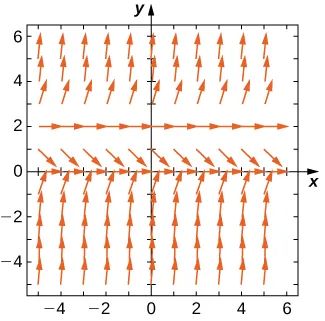70.

$y ( 0 ) = 3 y ( 0 ) = 3$

71.

$y ( 0 ) = 1 y ( 0 ) = 1$

72.

$y ( 0 ) = −1 y ( 0 ) = −1$

73.

Are there any equilibria? What are their stabilities?

Draw the direction field for the following differential equations, then solve the differential equation.

74.

$y ′ = t 3 y ′ = t 3$

75.

$y ′ = e t y ′ = e t$

76.

$d y d x = x 2 cos x d y d x = x 2 cos x$

77.

$d y d t = t e t d y d t = t e t$

78.

$d x d t = cosh ( t ) d x d t = cosh ( t )$

Draw the directional field for the following differential equations. What can you say about the behavior of the solution? Are there equilibria? What stability do these equilibria have?

79.

$y ′ = y 2 − 1 y ′ = y 2 − 1$

80.

$y ′ = y − x y ′ = y − x$

81.

$y ′ = 1 − y 2 − x 2 y ′ = 1 − y 2 − x 2$

82.

$y ′ = t 2 sin y y ′ = t 2 sin y$

83.

$y ′ = 3 y + x y y ′ = 3 y + x y$

Match the direction field with the given differential equations. Explain your selections.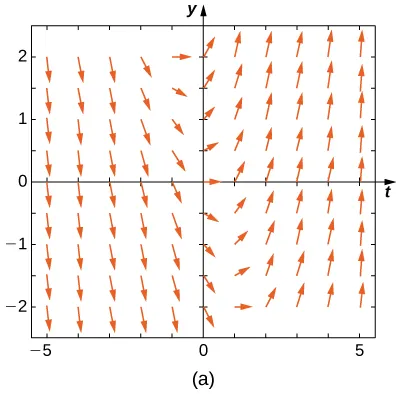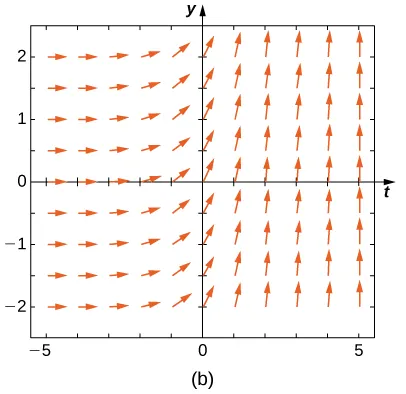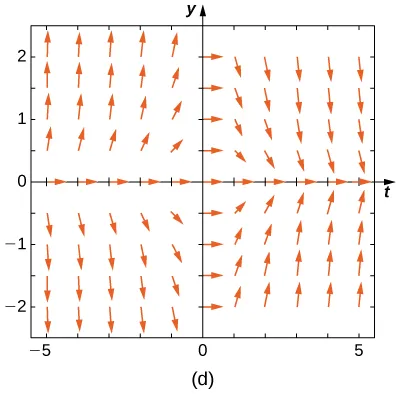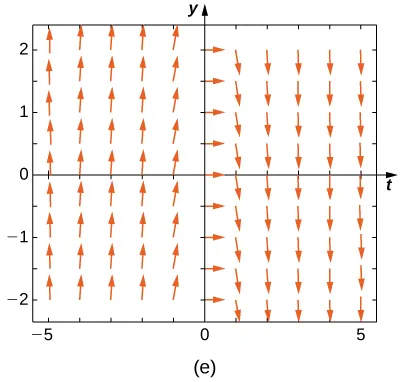84.

$y ′ = −3 y y ′ = −3 y$

85.

$y ′ = −3 t y ′ = −3 t$

86.

$y ′ = e t y ′ = e t$

87.

$y ′ = 1 2 y + t y ′ = 1 2 y + t$

88.

$y ′ = − t y y ′ = − t y$

Match the direction field with the given differential equations. Explain your selections.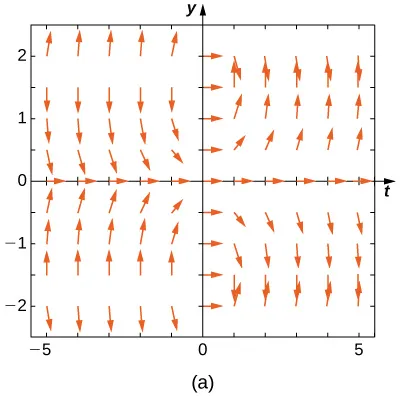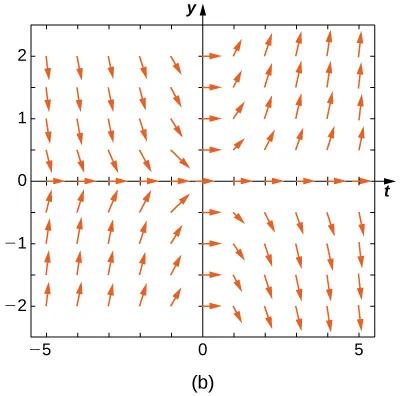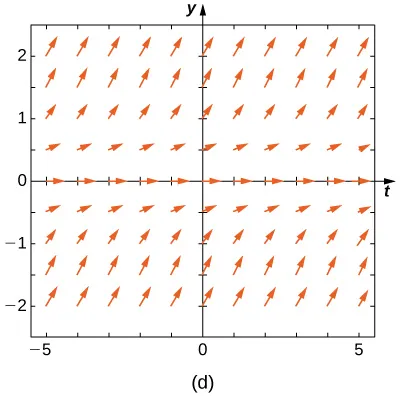89.

$y ′ = t sin y y ′ = t sin y$

90.

$y ′ = − t cos y y ′ = − t cos y$

91.

$y ′ = t tan y y ′ = t tan y$

92.

$y ′ = sin 2 y y ′ = sin 2 y$

93.

$y ′ = y 2 t 3 y ′ = y 2 t 3$

Estimate the following solutions using Euler’s method with $n=5n=5$ steps over the interval $t=[0,1].t=[0,1].$ If you are able to solve the initial-value problem exactly, compare your solution with the exact solution. If you are unable to solve the initial-value problem, the exact solution will be provided for you to compare with Euler’s method. How accurate is Euler’s method?

94.

$y ′ = −3 y , y ( 0 ) = 1 y ′ = −3 y , y ( 0 ) = 1$

95.

$y ′ = t 2 y ′ = t 2$

96.

$y′=3t−y,y(0)=1.y′=3t−y,y(0)=1.$ Exact solution is $y=3t+4e−t−3y=3t+4e−t−3$

97.

$y′=y+t2,y(0)=3.y′=y+t2,y(0)=3.$ Exact solution is $y=5et−2−t2−2ty=5et−2−t2−2t$

98.

$y ′ = 2 t , y ( 0 ) = 0 y ′ = 2 t , y ( 0 ) = 0$

99.

[T] $y′=e(x+y),y(0)=−1.y′=e(x+y),y(0)=−1.$ Exact solution is $y=−ln(e+1−ex)y=−ln(e+1−ex)$

100.

$y′=y2ln(x+1),y(0)=1.y′=y2ln(x+1),y(0)=1.$ Exact solution is $y=−1(x+1)(ln(x+1)−1)y=−1(x+1)(ln(x+1)−1)$

101.

$y′=2x,y(0)=0,y′=2x,y(0)=0,$ Exact solution is $y=2x−1ln(2)y=2x−1ln(2)$

102.

$y′=y,y(0)=−1.y′=y,y(0)=−1.$ Exact solution is $y=−ex.y=−ex.$

103.

$y′=−5t,y(0)=−2.y′=−5t,y(0)=−2.$ Exact solution is $y=−52t2−2y=−52t2−2$

Differential equations can be used to model disease epidemics. In the next set of problems, we examine the change of size of two sub-populations of people living in a city: individuals who are infected and individuals who are susceptible to infection. $SS$ represents the size of the susceptible population, and $II$ represents the size of the infected population. We assume that if a susceptible person interacts with an infected person, there is a probability $cc$ that the susceptible person will become infected. Each infected person recovers from the infection at a rate $rr$ and becomes susceptible again. We consider the case of influenza, where we assume that no one dies from the disease, so we assume that the total population size of the two sub-populations is a constant number, $N.N.$ The differential equations that model these population sizes are

$S′=rI−cSIandI′=cSI−rI.S′=rI−cSIandI′=cSI−rI.$

Here $cc$ represents the contact rate and $rr$ is the recovery rate.

104.

Show that, by our assumption that the total population size is constant $(S+I=N),(S+I=N),$ you can reduce the system to a single differential equation in $I:I′=c(N−I)I−rI.I:I′=c(N−I)I−rI.$

105.

Assuming the parameters are $c=0.5,N=5,c=0.5,N=5,$ and $r=0.5,r=0.5,$ draw the resulting directional field.

106.

[T] Use computational software or a calculator to compute an approximate solution to the initial-value problem $y ′ = t y , y ( 0 ) = 2 y ′ = t y , y ( 0 ) = 2$ using Euler's Method with a given step size $h . h .$ Find the solution at $t = 1 t = 1$ in terms of $h . h.$ Hint: Here is "pseudo-code" for how to write a computer program to perform Euler's Method for $y ′ = f ( t , y ) , y ( 0 ) = y 0 : y ′ = f ( t , y ) , y ( 0 ) = y 0 :$

• Create function $f ( t , y ) . f ( t , y ) .$

• Define parameters $y ( 0 ) = y 0 , t ( 0 ) = 0 , y ( 0 ) = y 0 , t ( 0 ) = 0 ,$ step size $h , h ,$ and total number of steps, $N N$

• Write a for loop:

for $k = 0 to N k = 0 to N$

$fn = f ( t ( k ) , y ( k ) ) fn = f ( t ( k ) , y ( k ) )$

$y ( k+1 ) = y ( k ) + h*fn y ( k+1 ) = y ( k ) + h*fn$

$t ( k+1 ) = t ( k ) + h t ( k+1 ) = t ( k ) + h$

Refer to your answer for Exercise 4.106 to solve the following exercises.

107.

Solve the initial-value problem for the exact solution.

108.

Draw the directional field

109.

Compute using $h=1h=1$

110.

Compute using [T] $h=0.1h=0.1$

111.

Compute using [T] $h=0.001h=0.001$

112.

Compute using [T] $h=0.0001h=0.0001$

113.

[T] Evaluate the exact solution at $t=1.t=1.$ Make a table of errors for the relative error between the Euler’s method solution and the exact solution. How much does the error change? Can you explain?

Consider the initial-value problem $y′=−2y,y(0)=2.y′=−2y,y(0)=2.$

114.

Show that $y=2e−2xy=2e−2x$ solves this initial-value problem.

115.

Draw the directional field of this differential equation.

116.

[T] By hand or by calculator or computer, approximate the solution using Euler’s Method at $t=10t=10$ using $h=5.h=5.$

117.

[T] By calculator or computer, approximate the solution using Euler’s Method at $t=10t=10$ using $h=100.h=100.$

118.

[T] Plot exact answer and each Euler approximation (for $h=5h=5$ and $h=100)h=100)$ at each $hh$ on the directional field. What do you notice?

Order a print copy

As an Amazon Associate we earn from qualifying purchases.